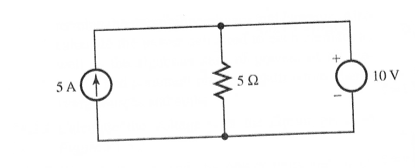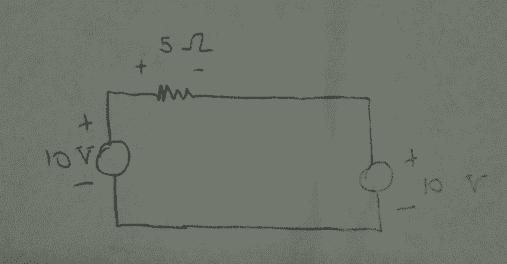# Circuits: Show that Power is Conserved

## Homework Statement

Show that conservation of power is satisfied:???

## The Attempt at a Solution

I am a little lost on this one. It should be straightforward, but I don't know what the approach is. Any thoughts on what technique I use to start this? I know that some of the current from the ideal source flows through the resistor and some through the voltage source (or sink?), but I am not sure how to determine how much?

Is it true to say that since the voltage sink (I am pretty sure it is a sink due to polarities of resistor and voltage element) that they must share voltage? I think that is the hint my professor gave, but I thought that voltage divides across resistors in parallel and not necessarily a resistor and generic sink in parallel.

Thanks.

## Answers and Replies

Mentor
The voltage across the resistor and current source is constrained by the voltage supply. So you can determine the current through the resistor. That should tell you where the rest of the current from the 5A source must go. You've then got voltages and currents for all components. Find the power sourced or sunk by each.

The voltage across the resistor and current source is constrained by the voltage supply. So you can determine the current through the resistor. That should tell you where the rest of the current from the 5A source must go. You've then got voltages and currents for all components. Find the power sourced or sunk by each.

Hello gneillUnfortunately I do not follow your reply. I have a 10 V source, a 5 Ω resistor (sink), and a 5A source. I do not know how to find the current through the resistor? I am assuming I need to use Ohms Law, but I don't know the voltage drop across the resistor? I mean I know that it is 10 V, but I don't understand why? How can I systematically compute the the drop across the resistor?

And has for the current source, I really don't know how to get a voltage out of that?

Thanks for your patienceI am really awful at circuits.

Mentor
The ideal voltage source will produce or sink any amount of current required in order to keep its voltage constant. Similarly, the ideal current supply will produce any amount of voltage required in order to keep its current constant.

The voltage drop across the resistor is constrained to be 10V by the 10V supply. It cannot be anything else. Similarly, the voltage across the current supply *must* be 10V, it too cannot be anything else.

If you have the voltage across the resistor then you know what the current through the resistor *must* be.

The ideal voltage source will produce or sink any amount of current required in order to keep its voltage constant. Similarly, the ideal current supply will produce any amount of voltage required in order to keep its current constant.

The voltage drop across the resistor is constrained to be 10V by the 10V supply. It cannot be anything else. Similarly, the voltage across the current supply *must* be 10V, it too cannot be anything else.

If you have the voltage across the resistor then you know what the current through the resistor *must* be.

I still don't get it ... I know, I know, I'm pretty slow. But let's take a detour for a moment: It seems as though I could take that current source parallel with that resistor and call it a Norton equivalent of some other imaginary circuit. As such, it has a current value of INo = 5A and a resistance of RNo = 5Ω. Hence, it can be replaced by a Thevenin equivalent voltage in series with a Thevenin resistance, where RTH = 5Ω and VTH = INoRTH = 10V.But there is something off about this. I am not sure what though. I still can't see how to find the powers...

Mentor
The Thevenin voltage would be 5A * 5Ω = 25V .

Edit: So what would be the resulting current in the circuit?

The Thevenin voltage would be 5A * 5Ω = 25V .

Haha .. sometimes I know how to multiplyEdit: So what would be the resulting current in the circuit?

Using KCL: 10V - 25V + Ickt*5Ω = 0 → Ickt = 3A.

Yay! So I can find the voltage across the resistor and show that conservation of energy is satisfied. But how do I show the power of the 10V and 25V elements? Is it possible? I know that cons of energy is good enough, but ...

Mentor
Careful. That Thevenin resistance is not your original resistor in the same place in the circuit. It may have the same value (5Ω) but it's not the same resistor!

You already know what the current must be in the original 5Ω resistor. It *must* have 10V across it. It's 5Ω. So the current *must* be....

Careful. That Thevenin resistance is not your original resistor in the same place in the circuit. It may have the same value (5Ω) but it's not the same resistor!

You already know what the current must be in the original 5Ω resistor. It *must* have 10V across it. It's 5Ω. So the current *must* be....

Doh! You lost me! What current are you asking about? For the circuit in the figure of post #5? Or something else?

Mentor
Doh! You lost me! What current are you asking about? For the circuit in the figure of post #5? Or something else?

The 5Ω resistor in post #1 is not the same as your 5Ω resistor in post #5. You cannot know if they will end up carrying the same current (in fact, they certainly will not in this case!).